# Test: Reaction Mechanism Level - 7

## 15 Questions MCQ Test Organic Chemistry | Test: Reaction Mechanism Level - 7

Description
Attempt Test: Reaction Mechanism Level - 7 | 15 questions in 30 minutes | Mock test for Chemistry preparation | Free important questions MCQ to study Organic Chemistry for Chemistry Exam | Download free PDF with solutions
*Answer can only contain numeric values
QUESTION: 1

### Among the following, the number of reaction(s) that produce(s) benzaldehyde is: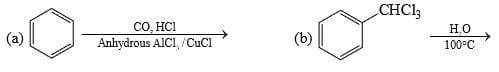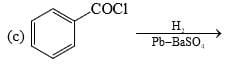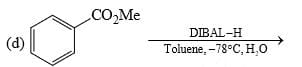Solution:

All four reactions produce benzaldehyde.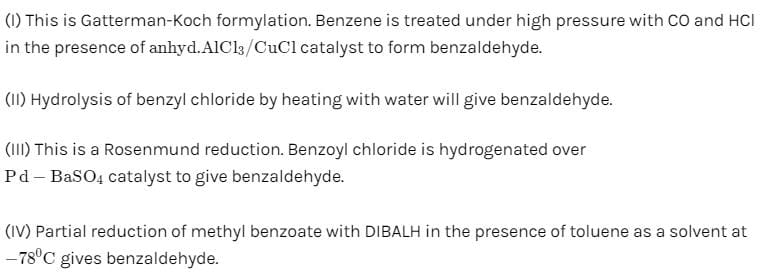*Answer can only contain numeric values
QUESTION: 2

### The total number of carboxylic acid groups in the product P is: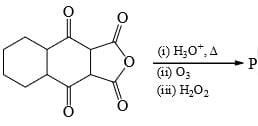Solution:
*Answer can only contain numeric values
QUESTION: 3

### In the scheme given below, the total number of intramolecular Aldol condensation products formed from ‘Y’ is: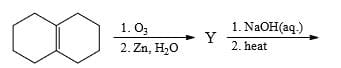Solution:
*Answer can only contain numeric values
QUESTION: 4

Amongst the following, the total number of compounds soluble in aqueous NaOH is: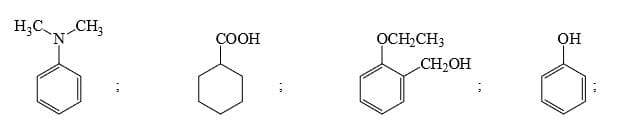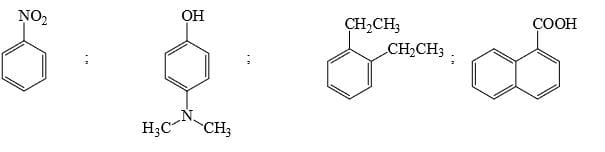Solution:
*Answer can only contain numeric values
QUESTION: 5

Among the following, how many compounds can form most stable hydrates: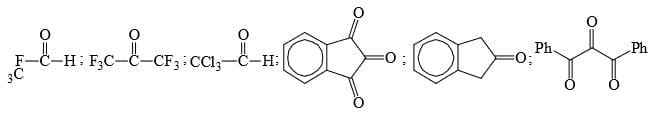Solution:
*Answer can only contain numeric values
QUESTION: 6

Identify total number of SN1 reactions: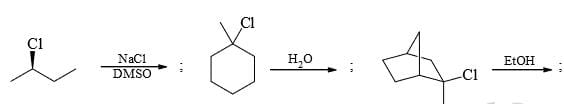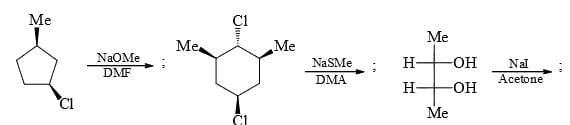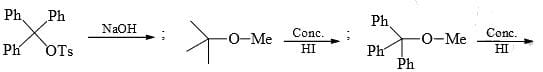Solution:
*Answer can only contain numeric values
QUESTION: 7

Identify total number of ‘β’-elimination reactions: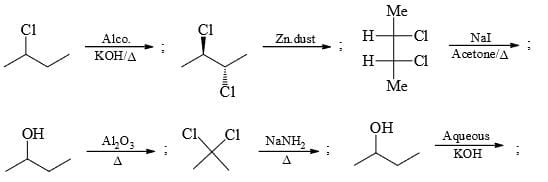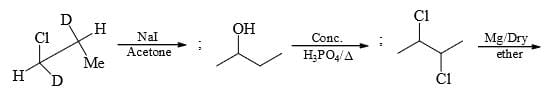Solution:
*Answer can only contain numeric values
QUESTION: 8

Calculate percentage of SN1 product if (R)-2-chloro butane on reaction with NaOH/H2O and acetone gives 98 per cent inverted product.

Solution:
*Answer can only contain numeric values
QUESTION: 9

Calculate total number of alkene products when 2-chloro-2-cyclobutyl hexane react with alcoholic KOH and heat.

Solution:
*Answer can only contain numeric values
QUESTION: 10

Calculate total number of α-H present in alkene formed when, 2,3-dimethyl butanol react with concentrated H2SO4/Δ.

Solution:
*Answer can only contain numeric values
QUESTION: 11

For the given sequence of reaction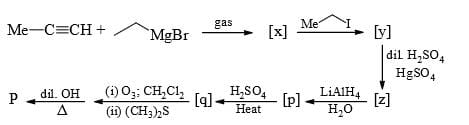How many products are obtained finally?

Solution: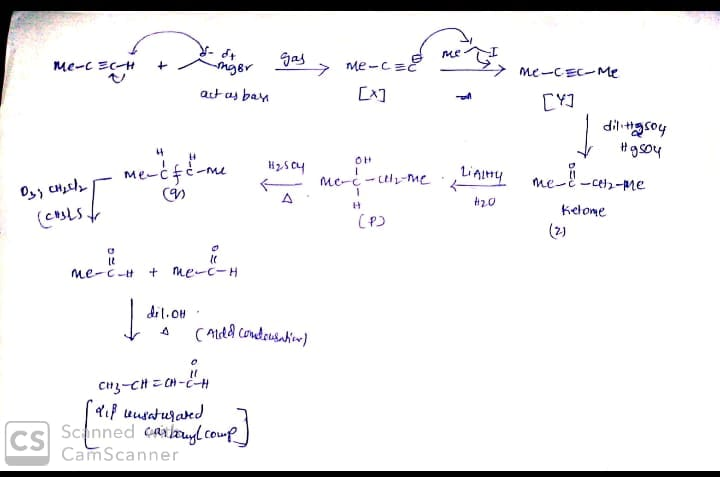*Answer can only contain numeric values
QUESTION: 12

Identify the total number of compounds that give positive test with Tollen’s reagent: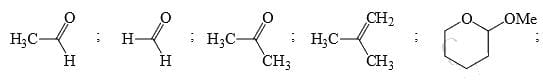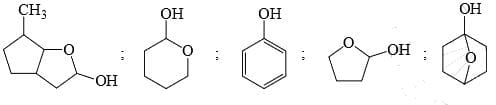Solution:
*Answer can only contain numeric values
QUESTION: 13

Identify the total number of compounds that give positive Iodoform test: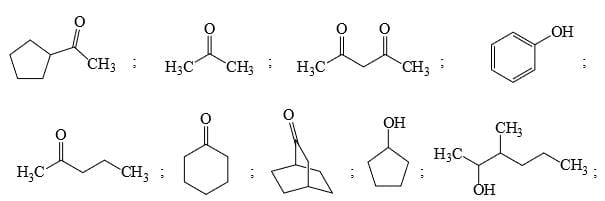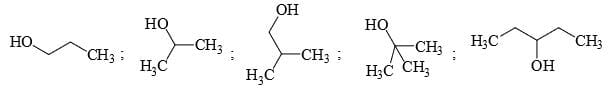Solution:
*Answer can only contain numeric values
QUESTION: 14

Identify the total number of compounds that give diastereomeric product on reaction with MeMgCl.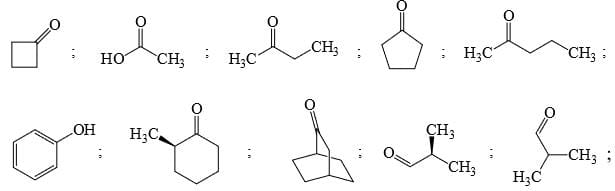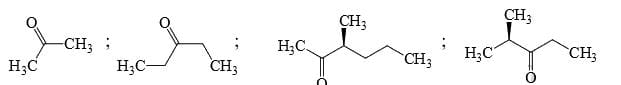Solution:
*Answer can only contain numeric values
QUESTION: 15

Identify that compounds that give Cannizaro reaction: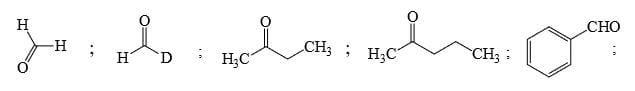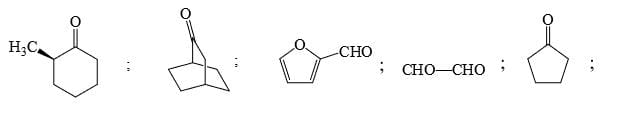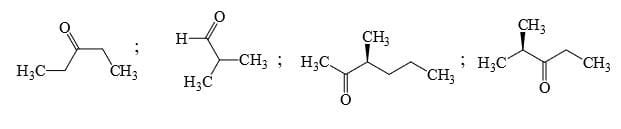Solution:Use Code STAYHOME200 and get INR 200 additional OFF Use Coupon Code